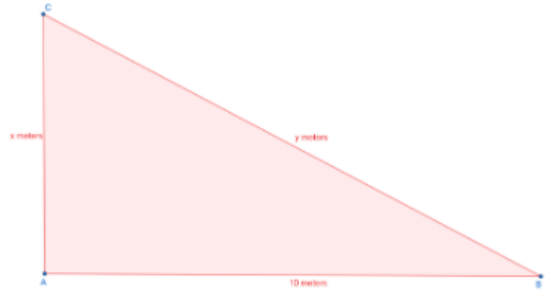QuestionAnswers

# A tree breaks due to the storm and the broken part bends so that the top of the tree touches the ground making an angle of $30^\circ$ with the ground. The distance from the foot of the tree to the point where the top touches the ground is 10 meters. Find the height of the tree.Verified
148.2k+ views
Hint: Use trigonometric ratios to find the unknown sides in the triangle and evaluate the equations to find the height of the tree.

First, let us draw a diagram to represent what’s given in the question.AC is the broken base of the tree and BC is the tree which has bent and touched the ground making an angle of $30^\circ$ with the ground.
(AC+BC) is the total height of the tree which is asked.
AB is the distance between the foot of the tree and the point where the tree touches the ground.
AB=10 meters
Let AC=x meters and let BC=y meters
$\angle ABC = 30^\circ ,\angle CAB = 90^\circ$
Let us find AC and BC using trigonometric ratios.
To find AC, we will find $\tan 30^\circ$. The value of $\tan \theta = \dfrac{{opposite}}{{adjacent}}$

$\tan 30^\circ = \dfrac{{AC}}{{AB}} \\ \dfrac{1}{{\sqrt 3 }} = \dfrac{x}{{10}} \\ x = \dfrac{{10}}{{\sqrt 3 }} \\$
Hence, $AC = \dfrac{{10}}{{\sqrt 3 }}$meters
To find BC, we will find $\cos 30^\circ$. The value of $\cos \theta = \dfrac{{adjacent}}{{hypotenuse}}$
$\cos 30^\circ = \dfrac{{AB}}{{BC}} \\ \dfrac{{\sqrt 3 }}{2} = \dfrac{{10}}{y} \\ y = \dfrac{{20}}{{\sqrt 3 }} \\$
Hence,$BC = \dfrac{{20}}{{\sqrt 3 }}$meters
Height of the tree= (AC+BC)
$= \dfrac{{10}}{{\sqrt 3 }} + \dfrac{{20}}{{\sqrt 3 }} \\ = \dfrac{{30}}{{\sqrt 3 }} \\ = \dfrac{{3 \times 10}}{{\sqrt 3 }} \\ = 10\sqrt 3 \\$
Hence, the total height of the tree is $10\sqrt 3$meters.

Note: Students tend to make the mistake of not adding AC and BC. Instead they give the answer as only the value of AC. The height of the tree means, before the tree was broken.